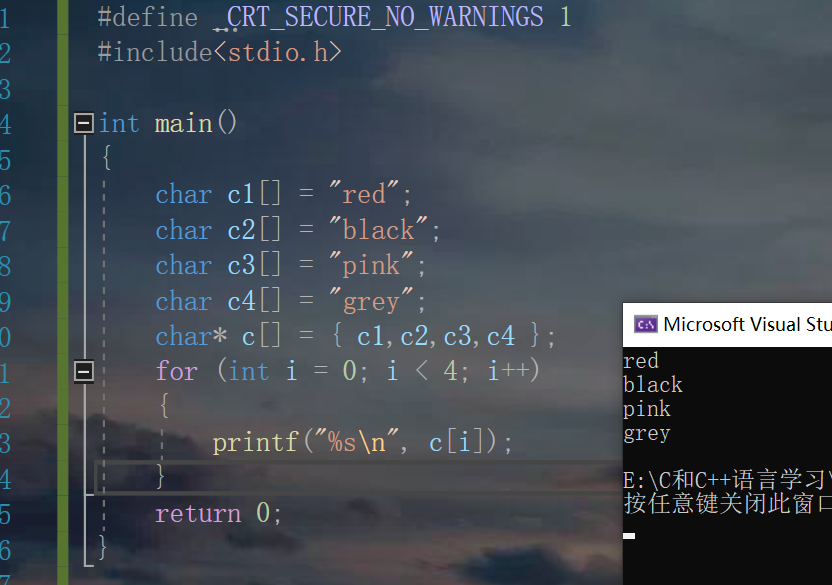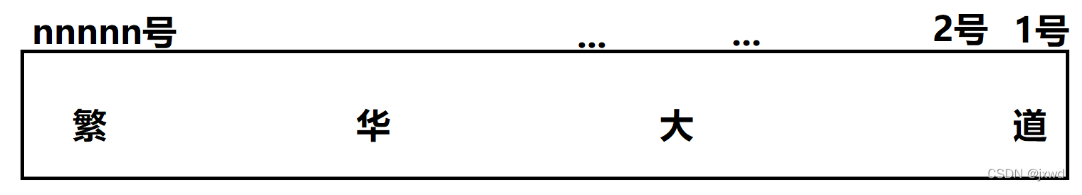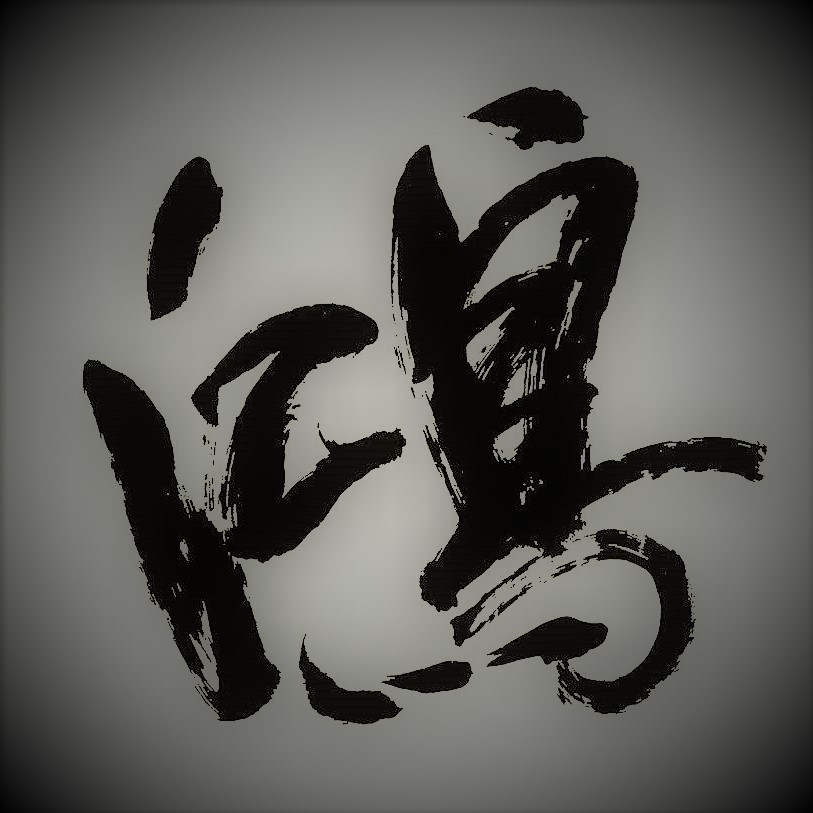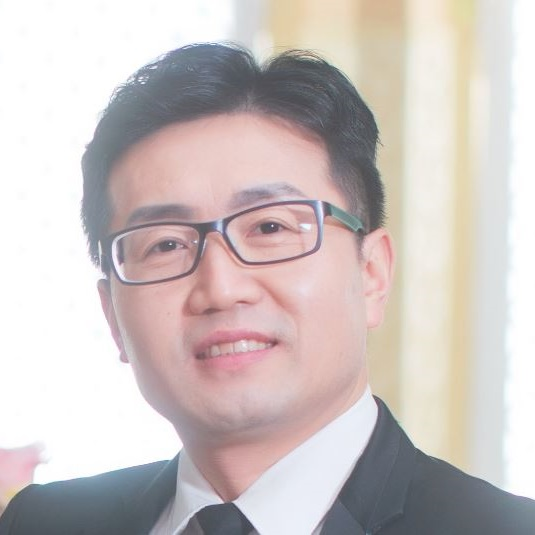+关注继续查看

@[toc]

# 学生时代所学的一些 C 语言知识点回顾（2）——指针

## 二 一些例子

# include<stdio.h>

int main(int argc,char *argv[]){
int i = 10, j, *p, *q, *x = &i;
p = &i;
q = p;
j = *&i;
printf("p = %d\n",*p);
printf("q = %d\n",*q);
printf("x = %d\n",*x);
printf("j = %d\n",j);
scanf("%d",&i);
printf("p = %d\n",*p);
printf("q = %d\n",*q);
printf("x = %d\n",*x);
printf("j = %d\n",j);
return 0;
}
/*
p = 10
q = 10
x = 10
j = 10
98
p = 98
q = 98
x = 98
j = 10
*/

*&i 对变量 i 使用 & 运算符产生指向指针变量的指针，对指针使用 * 运算符则可以返回到原始变量。

# include<stdio.h>

int main(int argc,char *argv[]){
int *p, *q, i = 989, j = 2019;
p = &i;
q = &j;
q = p;
printf("p = %d\n",*p);
printf("p : %p\n",p);
printf("j = %d\n",j);
printf("q = %d\n",*q);
printf("q : %p\n",q);
return 0;
}
/*
p = 989
p : 0x7fff51751e5c
j = 2019
q = 989
q : 0x7fff51751e5c
*/

# include<stdio.h>

int main(int argc,char *argv[]){
int *p, *q, i = 989, j = 2019;
p = &i;
q = &j;
*q = *p;
printf("p = %d\n",*p);
printf("p : %p\n",p);
printf("j = %d\n",j);
printf("q = %d\n",*q);
printf("q : %p\n",q);
return 0;
}
/*
p = 989
p : 0x7ffc00d359ac
j = 989
q = 989
q : 0x7ffc00d359a8
*/

# include<stdio.h>

int main(int argc,char *argv[]){
char i, *p;
p = &i;
scanf("%c",p);
printf("p = %c\n",*p);
printf("i = %c\n",i);
return 0;
}
/*
e
p = e
i = e
*/

# include<stdio.h>

int main(int argc,char *argv[]){
int a, b;
int *max(int *,int *);
scanf("%d%d",&a,&b);
printf("The max number is: %d\n",*max(&a,&b));
return 0;
}

int *max(int *a, int *b){
*a = *a + 5;
if (*a > *b)
return a;
else
return b;
}
/*
1 4
The max number is: 6
*/

# include<stdio.h>

int main(int argc,char *argv[]){
int a, b;
int max(int *,int *);
scanf("%d%d",&a,&b);
printf("The max number is: %d\n",max(&a,&b));
return 0;
}

int max(int *a, int *b){
*a = *a + 5;
if (*a > *b)
return *a;
else
return *b;
}
/*
1 4
The max number is: 6
*/

# include<stdio.h>

int main(int argc,char *argv[]){
int x = 2019, y=2023;
int *a = &x, *b = &y, c;
c = (*a + *b) * 2 + 20;
printf("Sum: %d\n",c);
return 0;
}
// Sum: 8104

# include<stdio.h>

int main(int argc,char *argv[]){
int p;
int example(int *);
scanf("%d",&p);
printf("%d\n",example(&p));
return 0;
}

int example(int *p){
int a = 10086;
printf("The original value is: %d\n",*p);
p = &a;
return *p;
}

# include<stdio.h>

int main(int argc,char *argv[]){
int p;
int example(int *);
scanf("%d",&p);
printf("%d\n",example(&p));
return 0;
}

int example(int *p){
printf("The original value is: %d\n",*p);
*p = 10086;
return *p;
}

# include<stdio.h>

int main(int argc,char *argv[]){
int p;
int example(const int *);
scanf("%d",&p);
printf("%d\n",example(&p));
return 0;
}

int example(const int *p){
int x = 10086;
printf("The original value is: %d\n",*p);
p = &x;
return *p;
}
/*
98
The original value is: 98
10086
*/

# include<stdio.h>

int main(int argc,char *argv[]){
int p;
int example(const int *);
scanf("%d",&p);
printf("%d\n",example(&p));
return 0;
}

int example(const int *p){
printf("The original value is: %d\n",*p);
*p = 10086;
return *p;
}

# include<stdio.h>

int main(int argc,char *argv[]){
int p;
int example(int * const);
scanf("%d",&p);
printf("%d\n",example(&p));
return 0;
}

int example(int * const p){
printf("The original value is: %d\n",*p);
*p = 10086;
// int x = 10086;
// p = &x;
return *p;
}
/*
98
The original value is: 98
10086
*/

# include<stdio.h>

int main(int argc,char *argv[]){
int p;
int example(const int * const);
scanf("%d",&p);
printf("%d\n",example(&p));
return 0;
}

int example(const int * const p){
printf("The original value is: %d\n",*p);
// *p = 10086;
// int x = 10086;
// p = &x;
return *p;
}
/*
98
The original value is: 98
98
*/

## 三 小结【C语言程序设计】知识点汇总4——指针相关
【C语言程序设计】知识点汇总4——指针相关
0 00基础C语言自学教程——第八节 函数指针数组的各种关系
const int* 是指向一个常量整数的指针，所以说，const int*所修饰的指针变量，其指针变量本身（即指向元素的地址）是可以被修改的，但是其指针所指向的值是不允许被修改的。
0 00基础C语言自学教程——第七节 初始指针

0 0【C++】如果你准备学习C++,并且有C语言的基础，我希望你能简单的过一遍知识点。
【C++】如果你准备学习C++,并且有C语言的基础，我希望你能简单的过一遍知识点。
0 0357 0603 0c语言基础学习07_关于指针的复习
============================================================================= 指针变量之间赋值是需要兼容的。 例如：int *a = int的地址char *b= char的地址--------------------------------------void类型的指针可以做任意类型地址的赋值操作。
764 0《C语言及程序设计》实践项目——链表初步

958 0576 01529844

C语言学习教程18833128

Go语言核心编程 - 数据结构和算法158147

Java编程入门131633066246

Java面试疑难点解析 - 面试技巧及语言基础314161

Go语言路上踩过的坑